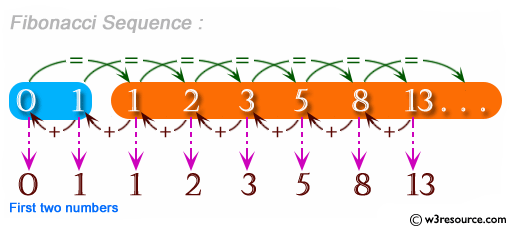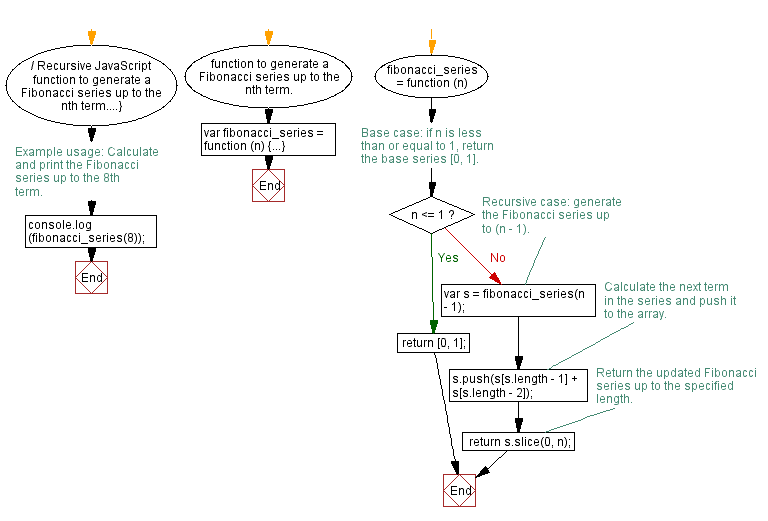# JavaScript: Get the first n Fibonacci numbers

## JavaScript Function: Exercise-6 with Solution

Write a JavaScript program to get the first n Fibonacci numbers.

Note: The Fibonacci Sequence is the series of numbers: 0, 1, 1, 2, 3, 5, 8, 13, 21, 34, . . . Each subsequent number is the sum of the previous two.

Pictorial Presentation:Sample Solution:-

HTML Code:

``````<!DOCTYPE html>
<html>
<meta charset="utf-8">
<title>Fibonacci Series</title>
<body>

</body>
</html>
```
```

JavaScript Code:

``````var fibonacci_series = function (n)
{
if (n===1)
{
return [0, 1];
}
else
{
var s = fibonacci_series(n - 1);
s.push(s[s.length - 1] + s[s.length - 2]);
return s;
}
};

console.log(fibonacci_series(8));
```
```

Output:

```[0,1,1,2,3,5,8,13,21]
```

Flowchart:Live Demo:

See the Pen javascript-recursion-function-exercise-6 by w3resource (@w3resource) on CodePen.

Improve this sample solution and post your code through Disqus

What is the difficulty level of this exercise?

Test your Programming skills with w3resource's quiz.

﻿

## JavaScript: Tips of the Day

Add padding to the beginning of a string

```const name = 'Minta Lofton';